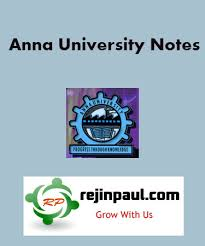-->

EE8251 Circuit Theory Syllabus Notes Question Paper Question Banks Anna University - Regulation 2017 2nd Semester Notes CBCS

Anna University Circuit Theory Syllabus Notes Question Bank Question Papers
Anna University EE8251 Circuit Theory Notes are provided below. EE8251 Notes all 5 units notes are uploaded here. here EE8251 Circuit Theory notes download link is provided and students can download the EE8251 Lecture Notes and can make use of it.EE8251 Circuit Theory Syllabus Regulation 2017
UNIT I BASIC CIRCUITS ANALYSIS
Resistive elements - Ohm’s Law Resistors in series and parallel circuits – Kirchoffs laws – Mesh current and node voltage - methods of analysis.
UNIT II NETWORK REDUCTION AND THEOREMS FOR DC AND AC CIRCUITS
Network reduction: voltage and current division, source transformation – star delta conversion.
Thevenins and Norton Theorems – Superposition Theorem – Maximum power transfer theorem – Reciprocity Theorem – Millman's theorem.
UNIT III TRANSIENT RESPONSE ANALYSIS
L and C elements -Transient response of RL, RC and RLC Circuits using Laplace transform for DC input and A.C. sinusoidal input.
UNIT IV THREE PHASE CIRCUITS
A.C. circuits – Average and RMS value - Phasor Diagram – Power, Power Factor and Energy.- Analysis of three phase 3-wire and 4-wire circuits with star and delta connected loads, balanced & un balanced – phasor diagram of voltages and currents – power measurement in three phase circuits.
UNIT V RESONANCE AND COUPLED CIRCUITS
Series and parallel resonance – their frequency response – Quality factor and Bandwidth - Self and mutual inductance – Coefficient of coupling – Tuned circuits – Single tuned circuits.

EE8251 Circuit Theory Syllabus Notes Question Bank with answers Anna UniversityReviewed by Anirudhan R on February 24, 2018 Rating: 5